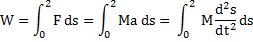# A body of mass 3 kg is under a force which causes a displacement in it, given by(in m). Find the work done by the force in 2 s in J is

## Question ID - 50932 :- A body of mass 3 kg is under a force which causes a displacement in it, given by(in m). Find the work done by the force in 2 s in J is

3537Next Question :

The average weight of 21 boys was recorded as 64 kg. if the weight of the teacher was added, the average increased by 1 kg. what was the teacher’s weight?

(a)86 kg                        (b) 64 kg                    (c) 72 kg                    (d)84 kg